HOME Electronics Directory Articles/ Tutorials eBooks About Us FORUM Links Contact Us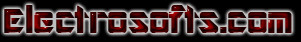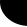Working with Digital Gates By L. R. Nagendra Prasad

Many designers need knowledge of basic digital circuits and logic. We were getting many feedbacks from readers who know the programming and want to have some idea of digital basics for interfacing or related works. We are working to bring some more articles on Boolean algebra and bitwise operations in programming. This is the first article in this series.

Logic gates forms the basic structural and functional part of the digital electronic circuits. Basically a logic gate is a circuit which

Ø       Combines two or more signals to give different signal of any logical or mathematical operation of the given signals.

Ø       Gives the complement or negative of the given signals.

Different types of logic gates available are

Inverter/NOT gate
AND gate
OR gate
NAND gate
NOR gate
XOR gate
XNOR gate

Normally a signal is called either HIGH or LOW in digital electronics. There are two logic conventions to give values of HIGH and LOW.

Positive logic: HIGH               1       (+5V)
LOW                0       (0V)

Negative logic: HIGH         0        (0V)
LOW         1        (+5V)

Hereafter we use positive logic in our discussion.

Inverter/NOT gate:

Symbol: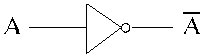Operation:

When a HIGH signal is given as input to an inverter, a LOW pulse will appear as output or vice-versa. The operation is summarized in truth table.

Truth table:

 Input A Output B 0 1 1 0

When to use:

Whenever a signal or pulse has to be inverted we use inverter/NOT gate.

Note:

The bubble indicated at the output of NOT gate is called negation indicator. When appearing on output, the bubble means that an internal ‘1’ produces an external ‘0’.

AND gate:

Symbol:Operation:

The AND gate performs logical multiplication more commonly known as AND function. It may have more than two inputs and only one output.

When all the inputs are HIGH, the output is HIGH.

When at least one of the inputs is LOW, the output is LOW.

Truth table:

 inputs output A B C 0 0 0 0 1 0 1 0 0 1 1 1

When to use:

To determine when certain conditions are simultaneously true, as indicated by HIGH levels.

Note:

We generally express the logical operation of a gate with a table that lists all input combinations and corresponding outputs. This table of combinations is called a truth table. When we have two inputs there are 4 combinations of inputs. Similarly if we have ‘n’ inputs we get ‘2n’ combinations.

OR gate:

Symbol:Operation:

The OR gate performs logical addition more commonly called OR function. It may have more than two inputs but only one output.

When at least one of the inputs is HIGH, output is HIGH.
When all the inputs are LOW, output is LOW.

Truth table:

 inputs output A B C 0 0 0 0 1 1 1 0 1 1 1 1

When to use:

To check whether at least one of the inputs is HIGH.

NAND gate:

Symbol:Operation:

The term NAND is a contraction of NOT-AND and implies an AND function with a complemented output.

When all the inputs are HIGH, the output is LOW.
When at least one of the inputs is LOW, the output is HIGH.

Truth table:

 inputs output A B C 0 0 1 0 1 1 1 0 1 1 1 0

Corollary:

The NAND is a very logic function because it is a “Universal Function” i.e. it can be used to construct an AND gate or an OR gate, an inverter or any combination of these functions.

Construction of AND, OR, NOT gates using NAND gate:

NOT gate: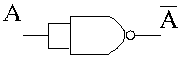Here both the inputs are same i.e. a, hence by the function of NAND we get a’ as output. This is also output of NOT gate.

AND gate:The output of NAND gate is given as two inputs to another NAND gate to get AB, the output of an AND gate.

OR gate:The OR can be implemented by use of NAND gates using basic Boolean Algebra.

When to use:

When there are different types of gates are required, all of them can be replaced by NAND gates only.

NOR:

Symbol:Operation:

The term NOR is a contraction of NOT-OR and implies an OR function with an inverted output.
When all the inputs are LOW, output is HIGH.
When at least one of the inputs is HIGH, output is LOW.

Truth table:

 inputs output A B C 0 0 1 0 1 0 1 0 0 1 1 0

Corollary:

Nor gate is also a universal gate because NOR function can be used to construct AND, OR, NOT or any combination of these functions.

NOT: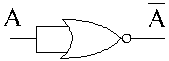OR:AND:When to use:

When there are different types of logic gates are required, they can be replaced by NOR gates.

XOR gate:

Symbol:Operation:

The acronym for XOR is Exclusive-Or. It is a widely used function because of special arithmetic properties. A special exclusive-or symbol,      is used.

When all the inputs are identical, HIGH/LOW, the output is LOW.
When the inputs have different logic levels, the output is HIGH.

Truth table:

 inputs output A B C 0 0 0 0 1 1 1 0 1 1 1 0

Use:

It is used in adder circuits, comparator circuits.

XNOR gate:

Symbol:Operation:

XNOR is a contraction of Exclusive-NOT-OR and implies a XOR function with an inverted output.
When all the inputs are identical, HIGH/LOW, the output is HIGH.
When all inputs have different logic levels, the output is LOW.

Truth table:

 inputs output A B C 0 0 1 0 1 0 1 0 0 1 1 1